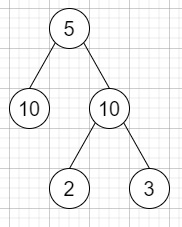# Equal Tree Partition in C++

Suppose we have a binary tree with n nodes, our task is to check whether it's possible to partition the tree to two trees which have the equal sum of values after deleting exactly one edge on the original tree.

So, if the input is likethen the output will be true.

• To solve this, we will follow these steps −

• Define one stack st

• Define a function solve(), this will take node,

• if node is null, then −

• return 0

• leftSum := solve(left of node)

• rightSum := solve(right of node)

• curr := val + leftSum + rightSum of node

• insert curr into st

• return curr

• From the main method do the following −

• solve(root)

• totalSum := top element of st

• delete element from st

• while (not st is empty), do −

• x := top element of st

• delete element from st

• y := totalSum - x

• if x is same as y, then −

• return true

• return false

## Example

Let us see the following implementation to get a better understanding −

Live Demo

#include <bits/stdc++.h>
using namespace std;
class TreeNode{
public:
int val;
TreeNode *left, *right;
TreeNode(int data){
val = data;
left = NULL;
right = NULL;
}
};
class Solution {
public:
stack <int> st;
int solve(TreeNode* node){
if (!node)
return 0;
int leftSum = solve(node->left);
int rightSum = solve(node->right);
int curr = node->val + leftSum + rightSum;
st.push(curr);
return curr;
}
bool checkEqualTree(TreeNode* root) {
solve(root);
int totalSum = st.top();
st.pop();
while (!st.empty()) {
int x = st.top();
st.pop();
int y = totalSum - x;
if (x == y)
return true;
}
return false;
}
};
main(){
Solution ob;
TreeNode *root = new TreeNode(5);
root->left = new TreeNode(10);
root->right = new TreeNode(10);
root->right->left = new TreeNode(2);
root->right->right = new TreeNode(3);
cout<<(ob.checkEqualTree(root));
}

## Input

TreeNode *root = new TreeNode(5);
root->left = new TreeNode(10);
root->right = new TreeNode(10);
root->right->left = new TreeNode(2);
root->right->right = new TreeNode(3);

## Output

1